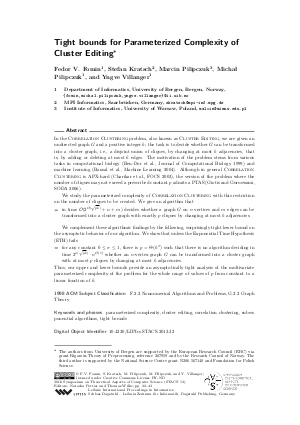Document# Tight bounds for Parameterized Complexity of Cluster Editing

### Authors Fedor V. Fomin, Stefan Kratsch, Marcin Pilipczuk, Michal Pilipczuk, Yngve Villanger## File

LIPIcs.STACS.2013.32.pdf
• Filesize: 0.64 MB
• 12 pages

## Cite As

Fedor V. Fomin, Stefan Kratsch, Marcin Pilipczuk, Michal Pilipczuk, and Yngve Villanger. Tight bounds for Parameterized Complexity of Cluster Editing. In 30th International Symposium on Theoretical Aspects of Computer Science (STACS 2013). Leibniz International Proceedings in Informatics (LIPIcs), Volume 20, pp. 32-43, Schloss Dagstuhl - Leibniz-Zentrum für Informatik (2013)
https://doi.org/10.4230/LIPIcs.STACS.2013.32

## Abstract

In the Correlation Clustering problem, also known as Cluster Editing, we are given an undirected graph G and a positive integer k; the task is to decide whether G can be transformed into a cluster graph, i.e., a disjoint union of cliques, by changing at most k adjacencies, that is, by adding or deleting at most k edges. The motivation of the problem stems from various tasks in computational biology (Ben-Dor et al., Journal of Computational Biology 1999) and machine learning (Bansal et al., Machine Learning 2004). Although in general Correlation Clustering is APX-hard (Charikar et al., FOCS 2003), the version of the problem where the number of cliques may not exceed a prescribed constant p admits a PTAS (Giotis and Guruswami, SODA 2006). We study the parameterized complexity of Correlation Clustering with this restriction on the number of cliques to be created. We give an algorithm that - in time O(2^{O(sqrt{pk})} + n+m) decides whether a graph G on n vertices and m edges can be transformed into a cluster graph with exactly p cliques by changing at most k adjacencies. We complement these algorithmic findings by the following, surprisingly tight lower bound on the asymptotic behavior of our algorithm. We show that unless the Exponential Time Hypothesis (ETH) fails - for any constant 0 <= sigma <= 1, there is p = Theta(k^sigma) such that there is no algorithm deciding in time 2^{o(sqrt{pk})} n^{O(1)} whether an n-vertex graph G can be transformed into a cluster graph with at most p cliques by changing at most k adjacencies. Thus, our upper and lower bounds provide an asymptotically tight analysis of the multivariate parameterized complexity of the problem for the whole range of values of p from constant to a linear function of k.
##### Keywords
• parameterized complexity
• cluster editing
• correlation clustering
• subexponential algorithms
• tight bounds

## Metrics

• Access Statistics
• Total Accesses (updated on a weekly basis)
0